# Discrete vs. Continuous RVs (Random Variables)

• A discrete RV has a countable number of possible outcomes

• number of students present

• number of red marbles in a jar

• number of heads when flipping three coins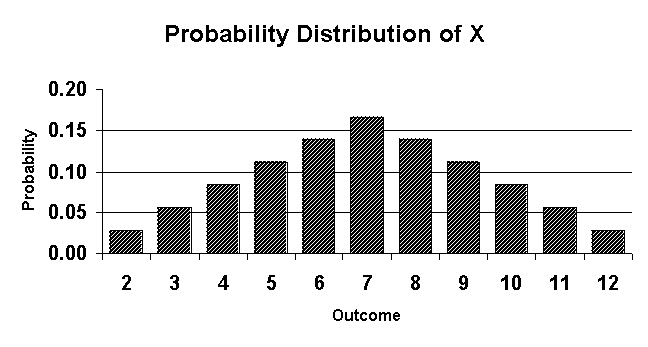• A continuous RV can take any values in an interval of numbers

• height of students in class

• weight of students in class

• time it takes to get to school

• distance traveled between classes# Probability Distribution

• The probability distribution of a discrete RV(X) list all the values possible and their probabilities• The probabilities must:

• All be a number between 0 and 1

• Together add up to 1

# Mean = Expected Value

• Formula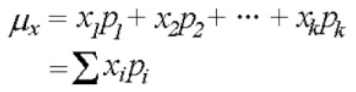• Calculator

• Type in X in L1 and P in L2• 1-Var Stats L1, L2

![П-В4 Pius Stver Editj(n ф TEns [NSTRUMENTS гоямдта ](./media/image110.png)

# Variance = Standard Deviation

• Formula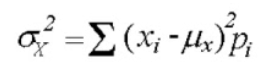• Calculator

![П-В4 Pius Stver Editj(n ф TEns [NSTRUMENTS гоямдта ](./media/image110.png)

# Practice Questions

• A bin contains ten \$1 bills, five \$2 bills, three \$5 bills, one \$10 bill, and one \$100 bill. A person is charged \$10 to select one bill. Let the random variable (X) be the amount someone wins by playing.

• Construct a probability distribution for these data.• What is the mean and standard deviation of the amount of money someone can expect to win?• You work for a company and are tasked with evaluating a proposed venture. The venture stands to make a profit of \$10,000 with probability 4/20, to make a profit of \$5200 with probability 8/20, to break even with probability 1/4, and to lose \$5000 with probability 3/20. The expected profit in dollars is? Would you recommend this venture?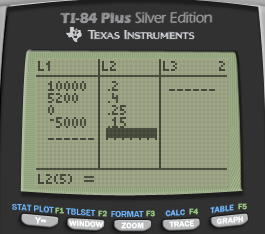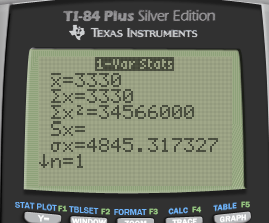• Answer: The expected profit is \$3330. Recommend, because the standard deviation is not so large